### The analysis of real data using a multiscale stochastic volatility model*

Warning: If your browser does not allow you to see the content of this website click here to download a word file that reproduces the material contained here.

1. Abstract

We use filtering and maximum likelihood methods to solve  a calibration problem for a multiscale  stochastic volatility model. The multiscale stochastic volatility model considered has been introduced in ,  and describes the dynamics of the asset price using as auxiliary variables two stochastic variances varying on two different time scales. Our aim  is to estimate the parameters of this  multiscale model (including the risk premium parameters when necessary) and its two initial stochastic variances from the knowledge, at discrete times, of the asset price  and, eventually, of the prices of call and/or put European options on the asset. This problem is translated in a maximum likelihood problem with the likelihood function defined through the solution of a filtering problem. The estimated values of the parameters and of the two initial stochastic variances are characterized as being a constrained maximizer of a likelihood  function. Furthermore we develop a  tracking procedure that is able to track the asset price and the values of its two stochastic variances  for   time values where there are no data available. The solution of the calibration problem and the tracking procedure are used to do the analysis of data time  series. Numerical  examples of the solution of the calibration problem and of the performance of the tracking procedure using synthetic and  real data are presented in  . The synthetic data time series  is analyzed using data samples made of observations done with a given time frequency. We consider observation frequencies ranging from “high” to “low”   and we study the performance of the calibration procedure as a function of the observation frequency. The real data studied are two time series of electric power price data taken from  the U.S. electricity market and the 2005 data relative to the US S&P 500 index and to the  prices of a call and a put European option on the S&P 500 index. In the study of real data we consider daily  data. The results obtained from the analysis of the synthetic and of the real data are very satisfactory.  Moreover in the real data case we use the estimated values of the parameters and of the initial stochastic variances obtained solving the calibration problem using a data time window to produce through the tracking procedure mentioned above  forecasts of the asset prices (electric power price or S&P 500 index), of the associated stochastic variances and of the option prices. The forecasts of the asset prices and of the option prices are compared with the prices actually observed. This comparison shows that the forecasts are of very high quality even when we consider “spiky” electric power price data. This website contains some auxiliary material including some animations that helps the understanding of  the work presented in . A more general reference to the work of the authors and of their coauthors in mathematical finance is the website: http://www.econ.univpm.it/recchioni/finance.

Acknowledgments:

· It is a pleasure to thank V. Kholodnyi and R. Whaley of Platts Analytics Inc. (Boulder, Colorado, USA) for providing us with the electric power price data.

· The numerical experience and data analysis reported in this website have been obtained using the computing resources of CASPUR (Roma, Italy) under contract: “Multiscale stochastic volatility models in finance and insurance” granted to the  Università di Roma “La Sapienza”. The support and  sponsorship of CASPUR are   gratefully acknowledged.

· The research reported in this website is partially supported by MUR – Ministero Università e Ricerca (Roma, Italy), 40%, 2007, under grant: “The impact of population ageing on financial markets, intermediaries and financial stability”. The support and sponsorship of MUR are gratefully acknowledged.

Keywords. Multiscale stochastic volatility models, filtering problem, calibration model, option pricing.

AMS (MOS) Subject Classification. 62F10, 35R30, 91B70.

M.S.C. classification. 34M50, 60H10, 91B28.

J.E.L. classification. C53, G12, C61.

2.  The multiscale stochastic volatility model, the filtering and calibration problems

For the convenience of the reader we summarize some results contained in . Let R and R+ be the sets of real and of positive real numbers respectively and t be a real variable that denotes time. Let St, t ≥0, be a stochastic process describing the asset (stock, commodity, index) price at time t≥0 and xt = log(St/S0), t ≥ 0, be the corresponding log-return. We associate to the asset price two stochastic variances described by the stochastic processes v1,t, v2,t, t ≥ 0, one fluctuating on a fast time scale and the other fluctuating on a long time scale. The dynamics of the stochastic process xt, v1,t, v2,t, t > 0, is defined by the following system of stochastic differential equations:where the quantities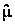,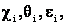ai, bi, i = 1,2, are real constants satisfying the following conditions: ci ³ 0, ei ³ 0, qi ³ 0,  bi  ≥ 0, i = 1,2. These conditions are due to the financial meaning of the constants.

The state variables  v1,t, v2,t , t>0, are the stochastic variances associated to xt, t>0,   Wt0,1, Wt0,2 ,Wt1, Wt2, t>0, are standard Wiener processes such that W00,1= W00,2=W01=W02=0,  dWt0,1, dWt0,2 ,dWt1, dWt2 are their stochastic differentials and we assume that  <dWt0,1dWt0,2 >= 0,  <dWt0,1dWt1 >= r0,1dt, <dWt0,2dWt2 >= r0,2dt,   <dWt1dWt2>=0 , where < × > denotes the mean of  × and r0,1, r0,2Î[-1,1] are  constants  known as correlation coefficients.   The fact that the model is a two scale stochastic volatility model is translated in the assumption that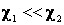. The equations (1), (2), (3)  must be equipped with an initial condition, that is:The random variables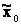,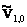,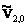are assumed to be concentrated in a point with probability one and we choose=0.  We  assume that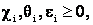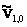,>0, i=1,2 and later in the multiscale model we will choose ai=-1/2, bi=1, i=1,2.

The model (1), (2), (3)  generalizes the Heston stochastic volatility model. In fact choosing a1 = -1/2, a2 = 0, b1 = 1, b2 = 0 the equations (1), (2) reduce to the Heston model and they become decoupled from equation (3). Remind that the stochastic variances and in particular the initial stochastic variances appearing in (5), (6) cannot be observed in real markets.

As already mentioned in all the numerical experiments presented here we use the multiscale model model and   we choose  in equations (1), (2), (3)  a1 = a2 = -1/2 and b1 = b2 = 1.  This choice translates in the multiscale model the usual relation between asset price, asset log-return and stochastic variance known from the Heston model. Remember that when the option prices are used as data of the calibration problem the discount rate r , that appears in the option price formulae, becomes a parameter that must be determined in the calibration, and in that case we will choose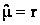in the option price formulae. More general problems where r is not necessarily equal toand ai, bi, i = 1,2, are parameters to be determined in the calibration can be studied. We will not consider them here.

Let us introduce the vector Q  =(e1,q1,r0,1,c1,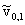,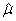,l1,e2,q2,r0,2,c2,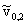,l2)  Î R13 where the parameters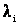, i=1,2, are the risk premium parameters that must be used when we consider option prices. The set M Ì R13  of the feasible vectors is defined as follows:The constraints that define M translate some elementary properties required to model (1), (2), (3).  Moreover, let ti, i = 0,1,¼,n, be time values such that ti < ti+1, i = 0,1,¼,n-1, without loss of generality we choose t0 = 0, and for later convenience we define tn+1 = +¥. Let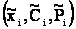be respectively the log-return of the asset price St and the prices of European vanilla call and put options on the asset having maturity time Ti (Ti > ti) and strike price Ki, observed at time t = ti, i = 0,1,¼,n. We suppose that the option prices observations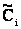,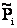are affected by a Gaussian error with mean zero and known variance fi, i = 0,1,¼,n,  and that the asset log-returns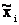, i = 0,1,¼,n, are observed without error. The filtering problem that we consider is the following:

Given the vector Q Î M find the probability density function p(x,v1,v2,t|Ft, Q), (x,v1,v2) Î R×R+×R+, t > 0, of the random variables xt, v1,t and v2,t, t > 0, solution of (1), (2), (3), (4), (5), (6), conditioned to the observations Ft = {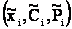: ti £ t, i > 0}, t > 0, and forecast the values of the state variables xt, v1,t, v2,t for t > 0 and in particular for t ¹ ti, i = 0,1,¼,n and for t > tn.

For t = t0 = 0 we define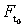= {= 0 }. Note that in the filtering problem stated above we do not make use of the option prices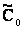,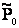observed at time t = t0. That is in the filtering problem at time t = t0 = 0, we use as datum only x0 == 0. In the filtering problem the initial stochastic variances, appearing in (5), (6) are assigned in the vector Q,  that  is supposed to be known, and can be considered as parameters. The call and put option prices,at time t = t0 = 0 will be used as data in the calibration problem. Many other filtering problems different from the one stated above that use as data different combinations of asset prices and/or option prices can be studied with simple extensions of the formulae that follows. We will not consider them here.

Let us define the following functions:

 pi(x,v1,v2,t| Q) = p(x,v1,v2,t|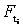, Q), (x,v1,v2) Î R×R+×R+, ti < t < ti+1, (x,v1,v2) Î R×R+×R+, ti < t < ti+1,   i = 0,1,¼, n,     (8)

to be the joint probability density functions of the random variables xt, v1,t, v2,t, solution (1), (2), (3), (4), (5), (6), when ti < t < ti+1 conditioned to the observationsmade up to time t = ti, i = 0,1,¼,n. The functions pi(x,v1,v2,t| Q), (x,v1,v2) Î R×R+×R+, ti < t < ti+1, i = 0,1,¼,n can be computed as solutions of a set of initial value problems for the Fokker Planck equation (see  and the reference therein) with suitable initial conditions that involve the knowledge of the option prices.

Using the functions pi(x,v1,v2,t| Q), (x,v1,v2) Î R×R+×R+, ti < t < ti+1, i = 0,1,¼, n, we can forecast the values of the state variables of the model xt, v1,t, v2,t, t > 0, respectively as the expected values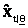,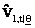,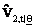, t > 0, conditioned to the observations contained in Ft, t > 0, of the random variables xt, v1,t, v2,t, t > 0, that is: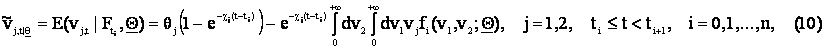where E(· | +) denotes the expected value of · conditioned to + and the functions fi(v1,v2;Q) are defined through the knowledge of the conditioned probability densities (8) (see  for further details) and are given as two-dimensional integrals of explicitly known integrands.  The formulae (9), (10) can be used to track the random variables xt, v1,t, v2,t, for t > 0, and in particular for t ¹ ti, i = 0,1,¼,n and for t > tn and they constitute the tracking procedure announced in the Abstract.

We note that the model (1), (2), (3) together with the associated option price formulae are parameterized by 13 real quantities, that is: the model parameters, ci, qi, ei, i = 1,2, the risk premium parameters li, i = 1,2, the correlation coefficients r0,i, i = 1,2, and the initial stochastic variances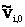, i = 1,2. That is, when we consider the  calibration problem using as data asset and option prices we use the vector Q  =(e1,q1,r0,1,c1,,,l1,e2,q2,r0,2,c2,,l2)  Î R13 and the set M Ì R13 defined in (10).

The vector Q Î R13 is the unknown of the calibration problem considered here when we use as data asset and option prices and the set M defines the set of the “feasible” vectors, that is the set of vectors satisfying the constraints. Later in the formulation of the calibration problem we will assume Q Î M. When we consider the filtering and calibration problems using as data only asset prices the risk premium parameters li, i = 1,2, that appear in the option price formulae, must be removed from the vector Q and the definition of the vector Q and of the set M must be changed consequently. To fix the ideas  we discuss in detail only the situation where asset prices and option prices are used as data in the filtering and in the calibration problem. We leave to the reader to work out the obvious changes that are needed when simpler problems are considered.

The calibration problem consists in determining the value of the vector Q Î M that makes most likely the observations, made at time t = ti, i = 0,1,¼,n. In the calibration problem we add to the data of the filtering problem the call and put option prices at time t = t0 and this fact improves greatly the quality of the estimates of the initial stochastic variances resulting from the solution of the calibration problem. The improvement of the quality of the estimated initial stochastic variances determines an improvement in the estimates of all the remaining parameters contained in the vector Q.

As suggested in ,  given the observationsat time t = ti, i = 0,1,¼,n,  we measure the (log-)likelihood of a vector Q Î M through the following (log-) likelihood function: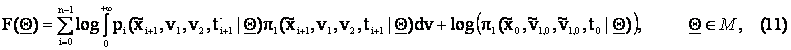where we have defined: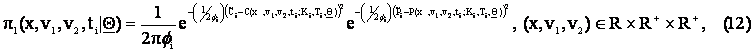and  pi(x,v1,v2,ti-| Q) =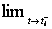pi(x,v1,v2,t| Q),means left limit for t that goes to ti, i = 1,2,¼,n and C and P are the formulae derived in  for the price of European vanilla call and put options in the multiscale model (1), (2), (3) respectively .  The calibration problem that we consider is the following one:

Given the observationsat time t = ti, i = 0,1,¼,n, determine the vector Q* Î M Ì R13 solution of the following nonlinear constrained optimization problem:

 max Q Î M F( Q).

(13)

Problem (23) is known as maximum (log-)likelihood problem since the vector Q* Î M solution of (13) is the vector that makes “more likely” the observations actually made. The vector Q* Î M is the solution of the calibration problem. Note that problem (13) is only one possible way of using the maximum likelihood method to formulate the calibration problem that we are considering. Many other formulations of the calibration problem are possible and legitimate.

3 Calibration problem and forecasting experiments using real data

In this section we show two experiments that use real data. In these two experiments daily data are used. The first experiment consists in the analysis of two time series of electric power price data, the first time series does not contain spikes while the second one contains spikes. No option prices are used as data in this experiment. The electric power price data studied are taken from the U.S. electricity market. This experiment shows that the calibration of the model on data containing spikes gives parameter estimates substantially different from the estimates obtained applying the calibration procedure to data that do not contain spikes. In particular the estimates of the speeds of mean reversion c1, c2 and of the volatilities of volatilities e1, e2 take different values depending on the presence or absence of spikes in the data. Using the solution of the calibration problem and the tracking procedure introduced in Section 2 we forecast electric power prices. We compare the electric power prices forecasted with the tracking procedure with the prices actually observed to establish the quality of the forecasted prices. This comparison shows that the forecasted prices are of very high quality. The second experiment shows how the calibration procedure works on the 2005 data relative to the S&P 500 index and to the price of European put and call options on this index. In this experiment using formulae (9), (10) and some other formulae we forecast the S&P 500 index and the option prices and the forecasted prices are compared to the historical data. This comparison establishes the high quality of the forecasted prices. Moreover we compare the results obtained with the maximum likelihood formulation of the calibration problem considered here with those obtained with the least squares formulation of the calibration problem studied in .

The first numerical experiment considers two time series of electric power price data. The first time series consists of 365 daily price observations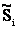i = 0,1,¼,364, that is it is a year, made of 365 days, of daily observations. The second time series consists of 765 daily price observations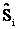, i = 0,1,¼764. In this experiment no option price data are used. Figure 1 shows the daily log-return increment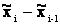=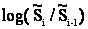, i = 1,2,¼,364, of the electric power price data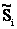, i = 0,1,¼,364. The time series shown in Figure 1 does not contain spikes. Figures 2 and 3 show the daily log-return increment of the second time series of electric power price data that is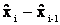=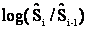, i=1,2,…,764. Figure 2 shows the daily log-return increment of the second time series of electric power price data in the first year of observation and Figure 3 shows the daily log-return increment in the second year of observation. The time series shown in Figures 2 and 3 contains spikes.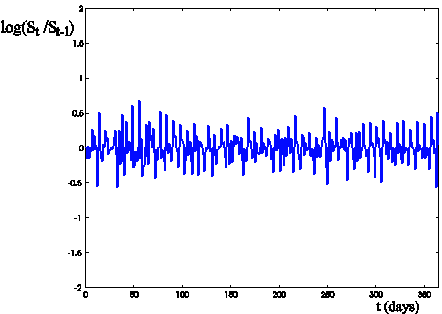Figure 1: Log-return increment log(St/St-1) of the electric power prices versus time t (days) (time series with no spikes, i=0,1,…,364, daily data).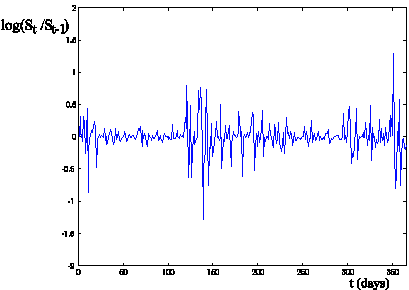Figure 2: Log-return increment log(St/St-1) of the electric power prices versus time t (days) (time series with spikes, i=0,1,2,…,364, first year of observation, daily data).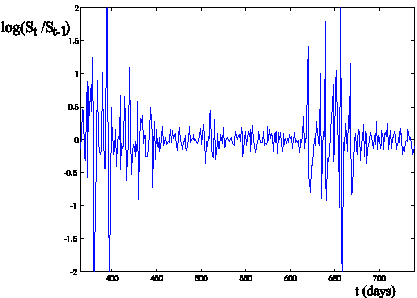Figure 3: Log-return increment log(St/St-1) of the electric power prices versus time t (days) (time series with spikes, i=365,366,…,764, second year of observation, daily data).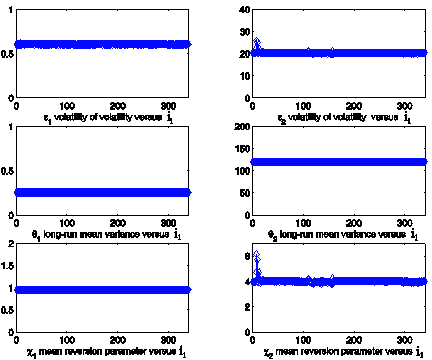Figure 4: Estimated parameter values obtained solving 340 calibration problems using as data  a data window of the electric power prices (time series with no spikes, Figure 1) versus the calibration problem number i1.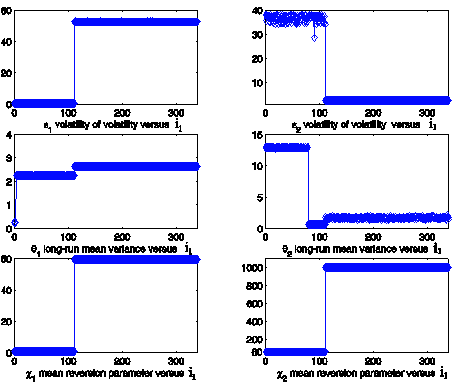Figure 5: Estimated parameter values obtained solving 340 calibration problems using as data a data window of the electric power prices (time series with spikes, Figure 2) versus the calibration problem number i1.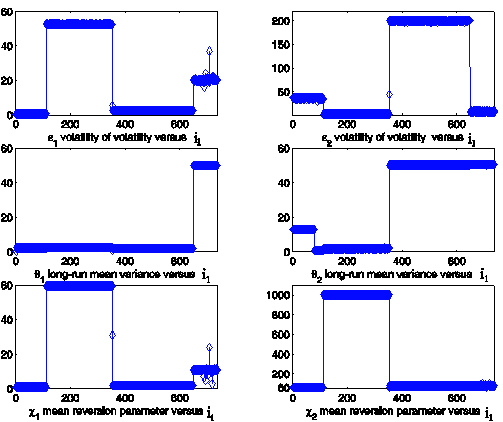Figure 6: Estimated parameter values obtained solving 740 calibration problems using as data a data window of the electric power prices (time series with spikes, Figures 2, 3) versus the calibration problem number i1.

We begin the analysis of these time series calibrating the multiscale model using a data  window made of 26 consecutive daily observations and we move this window along the time series substituting the first observation of the window with the next observation after the window. Using this procedure in the case of the time series shown in Figure 1 we solve 340 (340=365-26+1) calibration problems numbered with the index i1, i1 = 1,2,¼,340 and in the case of the time series shown in Figures 2 and 3 we solve 740(740=765-26+1) calibration problems numbered with the index i1, i1 = 1,2,¼, 740. The index i1 is the value of the index i associated to the first day contained in the data window used in the calibration. Figures 4 and 5 show the results obtained solving the calibration problems associated to the two time series of data as a function of the index i1 for i1 = 1,2,¼,340. Figure 4 shows the results obtained processing the time series of electric power price data with no spikes (shown in Figure 1). Remind that this time series is made of one year of daily data. Figure 5 shows the results obtained processing the first year of the time series of electric power price data with spikes (shown in Figure 2). We focus our attention on Figures 4, 5 to point out the different behaviour of the estimated parameter values in the two cases. In absence of spikes (Figure 4) the parameters ei, qi, ci, i = 1,2, are stable, that is they are approximately constants as a function of i1, and c1 and c2 are of the same order of magnitude (i.e.: c1 » 1, c2 » 4). In presence of spikes (Figure 5) the parameters ei, qi, ci, i = 1,2, have a jump in correspondence of the first spike. However comparing Figure 2 and Figure 5 we can see that the calibration procedure produces two values of the ratio c1/c2, in particular after the spike (i.e. i1 > 100) Figure 5 shows a jump in the ratio c1/c2 and we have c1 << c2.

Figure 6 shows the estimated values of the parameters ei, qi, ci, i = 1,2, during the entire observation period covered by the data shown in Figures 2 and 3 (time series with spikes). We note the presence of a jump in the estimated parameter values around i1 = 395. Note that when we solve the calibration problem corresponding to i1 = 395 we use as data the 26 consecutive daily observations starting with the 395th observation day. That is, this calibration problem takes as input data one of the spikes (see Figure 3) contained in the time series. The fact that Figures 4, 5, 6 show that the model parameters as a function of the index i1 are approximately piecewise constant functions suggests that the model and the calibration procedure proposed are well suited to establish a stable relationship between the data and the unknown of the calibration problem.

Moreover using formulae (9), (10), that is using the tracking procedure,  we forecast the electric power prices using as data of the calibration problems the data corresponding to the time series containing the spikes (shown in Figures 2, 3). That is we take a data window of 26 consecutive days in the time series of electric power price data we solve the corresponding calibration problem, we use the (approximate) solution of the calibration problem Q = Q* and formulae (9), (10) to forecast the values of the state variables in the future. The future consists in the time period after the last day whose observation is contained in the data window used in the calibration problem. The data window used as input data of the calibration problem is rolled through the time series using the procedure already described.

The results obtained  in this forecasting exercise are illustrated in Table 1, Figures 7, 8, Animation 1, Animation 2, Animation 3.

Table 1 establishes the quality of the forecasted values of the electric power log-returns when we do forecasts up to fifteen days in the future and we process the data corresponding to the time series shown in Figures 2, 3. That is Table 1 contains the mean value of the relative error,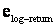, committed approximating the observed log-return with the forecasted log-return, the column “trend” of Table 1 contains (in percent) the number of times that the forecasted log-return moves in the correct direction with respect to the log-return of the first day contained in the data used in the calibration, that is increases when the historical log-return increases and decreases when the historical log-return decreases. The last column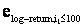of Table 1 contains the same quantity thancomputed on the log-returns observed before the first spike, that is for i1 £ 100. In Table 1 we consider forecasts 1, 2, 3, 4, 5 and 15 days in the futures, the quantities shown in the Table 1 show how the quality of the forecasts deteriorates when we go deeper into the future.

Finally Figures 7, 8  show the forecasted values of the log-return (one day in the future) and the time series of the log-returns corresponding to the time series shown in Figures 2, 3 as a function of time t (days). Figure 8 reproduces on a different scale the part of Figure 7 corresponding to 300 £ t £ 500. This is the region where severe spikes occur (see Figures 2, 3). Figures 7 and 8 show the very high quality of the (one day in the future) forecasted log-returns in a more convincing way than Table 1 where we consider highly aggregated performance indices. The Animations 1, 2, 3 show respectively the quality  of the forecasted log-returns one day, five days and fifteen days in the future.

 number of days in the futuretrend (%)1 0.0485 90.53% 0.0167 2 0.0786 82.27% 0.0268 3 0.0968 77.54% 0.0310 4 0.1078 73.88% 0.0324 5 0.1131 72.94% 0.0322 15 0.1773 63.06% 0.0474

Table 1: Mean relative errors and “trend” of the forecasted values of the electric power log-returns of the time series with spikes when compared to the log-returns actually observed.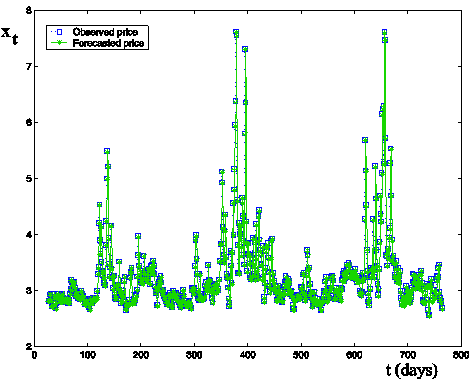Figure 7: Forecasted values (one day in the future) (stars) and observed values (squares) of the log-returns of electric power prices (time series with spikes, Figures 2, 3) versus time t (days).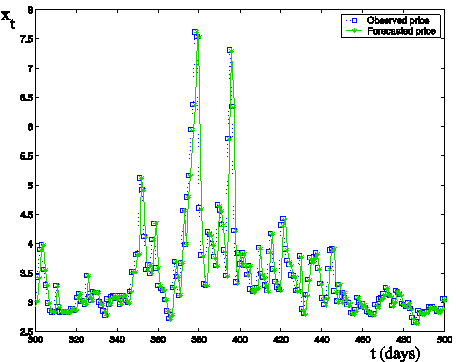Figure 8: Forecasted values (one day in the future) (stars) and observed values (squares) of the log-returns of electric power prices (time series with spikes, Figures 2,3) versus time t (days).

In all the animations the square is the observed value of the log-return and the star is the forecasted value.

Animation 1: Forecasted log-return one day in the future (data time series with spikes Figures 2, 3) click here, reduced animation on a different scale click here

Animation 2: Forecasted log-return five days in the future data time series with spikes Figures 2, 3) click here, reduced animation on a different scale click here

Animation 3: Forecasted log-return fifteen days in the future data time series with spikes Figures 2, 3) reduced animation on a different scale click here

The second numerical experiment consists in the analysis of the daily closing values of the S&P 500 index and of the daily bid prices of the European call and put options on the S&P 500 index with maturity date December 16, 2005 and strike price K = 1200 during the period of about eleven months that goes from January 3, 2005 to November 28, 2005. Due to the number of trading days in the year 2005 the time unit is a “year" made of 253 days. Note that the call and the put options mentioned above whose prices have been used as data in this experiment during the period January, November, 2005 had a significant volume traded. To avoid excessive numerical work we analyze in detail the market data (i.e. S&P 500 index and the European vanilla call and put option prices on this index) only in the months of January, February, May, June, October and November, 2005. The results presented are representative of the results obtainable from an exhaustive analysis of the eleven months of data available. We have solved the calibration problem (13) using the data contained in a window made of fifteen consecutive observation days, that is fifteen daily observations of the log-return of the S& P 500 index and of the call and put option prices on S& P 500 index having maturity time December 16, 2005 and strike price K = 1200. In this data window there are three data for each day of observation and fifteen days of observation, that is there are 45 data.

We solve three calibration problems, the first one uses as data the data relative to the first fifteen observation days of January 2005, the second one uses as data the data relative to the last twelve observation days of May 2005 and the first three observation days of June 2005, and the third one uses as data the data relative to the last twelve observation days of October 2005 and the first three observation days of November 2005. These three calibration problems have been chosen in order to be able to compare the results presented here with those presented in .  The three sets of estimated parameter values obtained solving these three calibration problems are used to forecast the value of the S&P 500 and the prices of its call and put European vanilla options one day, one week and one month in the future (that is one day, one week, one month after the day of the last observation contained in the data used in the calibration problem). The quality of these forecasts is established by comparison with the corresponding historical data. Moreover a comparison of the results obtained using the calibration procedure proposed here with the results obtained using the least squares formulation of the calibration problem proposed in  is presented.

The forecast of the S& P 500 index is done with the tracking procedure derived from (9), (10) already discussed. The forecast of the option prices is done using formulae derived in    and recalled in  starting from the model parameters, including the correlation coefficients, the risk premium parameters and the initial stochastic variances, estimated solving the calibration problem. We use option formulae  with two different choices of asset price, that is: the price actually observed in the day of the forecast or the asset price forecasted using formula (10). The first choice is considered in order to compare the results obtained in   and in this website with those presented in   where the asset price actually observed the day of the forecast was used. We note that in the option price formulae we use always the values of the stochastic variances obtained using formula (10). That is, given the stochastic variances v1,t, v2,t, at time t = t0 =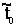whereis the time of the first observation used in the calibration problem (that is given the initial stochastic variances, i = 1,2 that are estimated solving the calibration problem) we forecast v1,t, v2,t, t > t0, using formula (10) where the vector Q is chosen to be the vector Q* obtained as solution of the calibration problem. Starting from the solution of the first calibration problem (data corresponding to the first fifteen observation days of January 2005) we forecast the index value and the option prices (in some specified days of January and February 2005). In Table 2 we show the relative errors made in the forecast of the S&P 500 index value eindex and of the bid prices of the European vanilla call option, ecall option, and put option, eput option, having strike price K = 1200 and maturity time T given by December 16, 2005 obtained forecasting up to a month in the future. In Table 2 the option prices are forecasted using as asset price the forecasted values of the S&P 500 index in the days of the forecasts. The errors are computed comparing the forecasted values with the historical data. The results shown in Table 2 relative to some specified days of January and February, 2005 are somehow representative of the results obtained with a more exhaustive analysis of the data available. Note that the forecasted values of the S&P 500 index are really satisfactory and of much higher quality than the forecasted values of the electric power prices (compare Table 1 and Table 2). This difference is due to two facts: the first one is the use of the option prices in the calibration procedure when we study the S& P 500 data. The option prices improve the quality of the solution of the calibration problem and of the conditioned probability densities. No option prices are used in the solution of the calibration problem for the electric power price data. The second one is the huge historical volatility of the time series of the electric power price data in comparison with the historical volatility of the S&P 500 index in 2005. This last fact makes the forecasting problem for the electric power price data substantially more difficult than the corresponding problem for the S&P 500 index in 2005.

 Number of days in the future eindex ecall option eput option 1 7.522·10-5 0.0659 0.0407 2 1.303·10-4 0.0559 0.0737 3 1.7509·10-4 0.0893 0.0952 4 2.6962·10-4 0.0528 0.0793 5 2.9114·10-4 0.0496 0.0822 15 3.7106·10-4 0.0268 0.1810 30 3.5094·10-4 0.0717 0.1099

Table 2: Relative errors of the forecasted values of the SP&500 and of the corresponding call and put option prices in some specified days of  January and February 2005 when compared to the prices actually observed. The model parameters employed have been obtained solving the calibration problem using as data the data relative to the first fifteen observation days of January 2005.

Finally we use the estimated model parameter vectors obtained as solution of the calibration problems to forecast the prices of all the European call options on the S&P 500 index traded in a given day. The days that we consider are January 28, 2005, June 7 and 28, 2005 and November 7, 14 and 28, 2005. Note that in the year 2005 we had approximately three hundreds European call options on the S&P 500 index traded. The forecast of the option prices of January 28, 2005 is done using the vector Q obtained solving the calibration problem using as data the first fifteen observation days of January 2005. The forecast of the option prices of June 7 and 28, 2005 and November 7, 14 and 28, 2005 are done using as data the first three observation days of the current month (June and November respectively) and the last twelve observation days of the previous month (May and October respectively). We consider the days January 28, June 7 and 28, November 7, 14 and 28, 2005 because we have at our disposal the results presented in  relative to the forecasts of the call option prices of these  days made using the model parameter vectors obtained as solution of the calibration problem formulated with the least squares procedure discussed in . We compare the call option prices obtained solving the calibration problem with the maximum likelihood procedure presented in this paper with those obtained solving the calibration problem with the least squares procedure introduced in . Let us introduce some notation necessary to illustrate the comparison. Let nobs be the number of call option prices available at time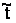and let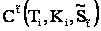,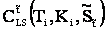and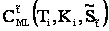denote respectively the (observed) prices at timeof European call options with strike price Ki and maturity time Ti, the prices generated using the appropriate formula (see , ) with the parameter vector obtained solving the calibration problem with the least squares procedure (LS) and with the parameter vector obtained solving the calibration problem using the maximum likelihood procedure (ML). The index i spans all the European call options traded at time. Note that nobs may depend on, we omit this dependence for simplicity. Let us define the  absolute error: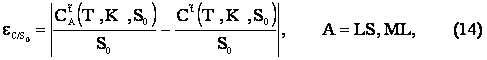obtained using the two calibration procedures (A = LS (least squares procedure), ML (maximum likelihood procedure)), in  we consider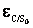as a function of the moneyness K/S0 = Ki/S0, i = 1,2,¼,nobs, where S0 =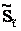is the value of the S&P 500 index at the transaction daywhere the option prices are forecasted and as a function of the time to maturity ti = Ti-, i = 1,2,¼, nobs.  Note that the sets of the values assumed by the moneyness and by the time to maturity may contain repeated values. In Table 3 we show the mean absolute error: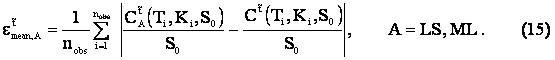Date t etmean, ML etmean,LS January 28, 2005 4.69 ·10-3 2.84·10-3 June 7, 2005 5.99 ·10-3 1.75·10-3 June 28, 2005 6.87 ·10-3 2.56·10-3 November 7, 2005 3.68·10-3 3.04·10-3 November 14, 2005 3.07·10-3 2.21·10-3 November 28, 2005 3.21·10-3 2.41·10-3

Table 3: Quality of the forecasted values of the option prices established comparing the forecasted prices with the prices actually observed when the maximum likelihood (ML) or the least squares (LS) method are used in the calibration of the multiscale model.

Note that the absolute errors shown  in Table 3 are referred to quantities roughly speaking of order 0.1.

We conclude this Section making some comments on the results obtained doing the forecasts with the least squares (LS) or with the maximum likelihood (ML) procedures.

We recall that in  we have solved three calibration problems using the least squares formulation and the call option price data of January 3, 2005, June 3, 2005 and November 3, 2005. Note that the least squares procedure to solve the calibration problem of January 3, 2005 uses nobs = 281 call option prices. Similarly the calibration problem of June 3, 2005 uses nobs = 281 call option prices and the calibration problem of November 3, 2005 uses the prices of nobs = 303 call options prices. We use the parameter estimated using the call option prices of January 3, 2005 (nobs = 281) to forecast the option prices of  January 28, 2005 (nobs = 258) and similarly we use the parameters estimated using the call option prices of June 3, 2005 (nobs = 281) to forecast the option prices of June 7, 2005 (nobs= 288) and  June 28, 2005 (nobs = 278) and the parameters estimated using the call option prices of November 3, 2005 (nobs = 303) to forecast the option prices of November 7, 2005 (nobs = 303), November 14, 2005 (nobs = 305) and November 28, 2005 (nobs = 292).

We proceed similarly with the parameter values estimated solving the three calibration problems mentioned above  with the maximum likelihood procedure. In fact we use the parameters estimated using the first fifteen daily observations of January 2005 of the S& P 500 index and of the prices of the European call and put options with K = 1200 and T given by December 16, 2005 to forecast the prices of all the call options traded on January 28, 2005. That is we forecast the prices of January 28, 2005 of nobs = 258 call options. Moreover we use the parameters estimated using the last twelve observation days of May 2005 and the first three observation days of June 2005 of the S& P 500 index and of the prices of the European call and put options with K = 1200 and T given by December 16, 2005 to forecast the prices of all the call options traded on June 7 and  June 28, 2005. That is we forecast the prices of June 7of nobs= 288 call options and the prices  of June 28, 2005 of nobs = 278 call options. Finally, we use the parameters estimated using the last twelve observation days of October 2005 and the first three observation days of November 2005 of the S& P 500 index and of the price of the European call and put options with K = 1200 and T given by December 16, 2005 to forecast the prices of all the call options traded on November 7, 14, 28, 2005. That is we forecast the prices of November 7, 2005 of nobs = 303 call options, the prices of November 14, 2005 of nobs = 305 call options and the prices of November 28, 2005 of nobs = 292 call options.

Table 3 shows the results obtained. Remind that in the forecasts considered in Table 3 we use as value of the S&P 500 index the actual value of the index the day of the forecast and not its forecasted value. The results presented in Table 3 show that the option prices forecasted with the two methods are approximately of the same quality. We can conclude that the maximum likelihood approach to the calibration problem has two advantages when compared to the least squares approach presented in . The first one is that a relatively small number of data is used to calibrate the model. In fact only one European call and one European put option prices and the corresponding value of the index are considered for each day of data and a time window of fifteen consecutive trading days is used as input data in the calibration problem. This corresponds to forty-five data. The least squares procedure uses as data all the call options traded in a given day that is approximately three hundred data. The second one is the fact that the maximum likelihood approach thank to the tracking procedure discussed previously allows to forecast the value of the asset, that is the value of the S&P 500 index. This is a consequence of the solution of the filtering problem. This makes possible to “forecast” option prices without knowing the value of the S&P 500 index the day of the forecast. Finally we note that going back to Table 2 we can say that the parameter values obtained using the maximum likelihood approach give satisfactory results when used to forecast the option prices up to a month in the future.

4. References

     F. Black, M. Scholes, “The pricing of options and corporate liabilities”, Journal of Political Economy, 81, 637-659 (1973).

     R. Cont, P. Tankov,  Financial modelling with jump processes, Chapman & Hall/CRC Financial Mathematics Series, Boca Raton, Florida, USA, 2004.

   L. Fatone,  F. Mariani, M.C. Recchioni, F. Zirilli: “The analysis of real data using a multiscale stochastic volatility model”, European Financial Management, 19(1), 153-179 (2013) .

   L. Fatone,  F. Mariani, M.C. Recchioni, F. Zirilli: “Calibration of a multiscale stochastic volatility model using as data European option prices”, Mathematical Methods in Economics and Finance, 3, 49-61 (2008).

  L. Fatone, F. Mariani, M.C. Recchioni, F. Zirilli: “European option prices under an explicitly solvable multi-scale stochastic volatility model and the analysis of the implied values of the parameters, of the correlation coefficients and of the initial stochastic variances of the model”, Journal of Futures Markets, 29(9), 862-893 (2009). (http://www.econ.univpm.it/recchioni/finance/w7).

    L. Fatone, F. Mariani, M.C. Recchioni, F. Zirilli: “The calibration of the Heston stochastic volatility model using filtering and maximum  likelihood  methods”,   in Proceedings of Dynamical Systems and Applications, G.S.Ladde, N.G.Medhin,Chuang Peng, M.Sambandham Editors,Dynamic Publishers,Atlanta,USA, 5, 170-181 (2008) (ISBN 1-890888-01-6).  (http://www.econ.univpm.it/recchioni/finance/w6).

       L. Fatone, F. Mariani, M.C. Recchioni, F. Zirilli: “Maximum likelihood estimation of the parameters of a system of stochastic differential equations that models the returns of the index of some classes of hedge funds”, Journal of Inverse and Ill-Posed Problems, 15, 329-362 (2007). (http://www.econ.univpm.it/recchioni/finance/w5  contains downloadable software).

     S.L. Heston, “A closed-form solution for options with stochastic volatility with applications to bond and currency options”, Review of Financial Studies, 6, 327-343 (1993).

 A. Lipton, Mathematical methods for foreign exchange, World Scientific Pubblishing Co. Pte. Ltd, Singapore, 2001.

        F. Mariani, G. Pacelli, F. Zirilli: "Maximum likelihood estimation of the Heston stochastic volatility model using asset and option   prices: an application of nonlinear filtering theory",  Optimization Letters, 2, 177-222 (2008),  (http://www.econ.univpm.it/pacelli/mariani/finance/w1  contains downloadable software).

Entry n. 2168